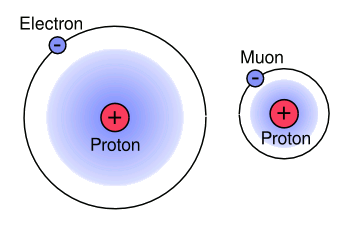### Proton Size Problem

March 20, 2013

The subatomic particle of the moment is the Higgs Boson, which apparently has been discovered in experiments at the Large Hadron Collider. More data needs to be forthcoming to confirm the spin of the particle detected (The Higgs would have a spin of zero), but it appears to be the particle predicted by the Standard Model. Having theory precede experiment is a good position. As Arthur Eddington once cautioned," It is also a good rule not to put overmuch confidence in the observational results that are put forward until they are confirmed by theory."

Far less exotic than the Higgs boson is the proton, discovered nearly a century ago by Ernest Rutherford. Over the course of that century, the proton was demoted from an elementary particle to a composite particle composed of three quarks (two up quarks and one down quark). Whereas an electron is quite nearly a point particle, the composite nature of the proton gives it a size.

As Rutherford's scattering experiments demonstrated, protons acts as if they were charged spheres. The edge of the sphere is not sharply defined, so the charge radius is taken as a root-mean-squared value. There have been many measurements of this charge radius, and the accepted value is 0.8768 femtometers (fm).

Until July, 2010, all such measurements were done using the interaction between protons and electrons, either by electron-proton scattering, or by spectroscopy of hydrogen atoms. electron-proton scattering was the first such measurement, and it was important enough that Robert Hofstadter shared the 1961 Nobel Prize in Physics.

The other method involves spectroscopy of the energy levels of neutral hydrogen. The neutral hydrogen atom consists of a nucleus of only one proton associated with a single electron, so it's a very simple system. These two independent measurements allowed a good estimate of the proton charge radius. While not as accurately determined as the value for other physical constants, it was known to within a percent.

All this certainty vanished in July, 2010, when a different sort of measurement showed a significantly smaller proton charge radius. As I wrote in a previous article (Fat Protons, July 12, 2010), measurements on an analog of the hydrogen atom in which a muon replaces the electron gave a proton charge radius of only 0.84184 fm.[2-5] This was five standard deviations from the established value. A repeat of this measurement has just yielded 0.84087 fm with a precision that places the established value seven standard deviations too high.[6-8]Proton radius values, as determined by different methods.

Note the small standard deviation bars for the muonic hydrogen data.

(Plotted with Gnumeric, using data from ref. .)

The measurements are done using pulsed laser spectroscopy of the Lamb shift of muon energy levels in muonic hydrogen. It's possible to calculate the proton radius using the measured Lamb shift. The Lamb shift, named after Nobel Physics Laureate, Willis Lamb, is the small difference in energy between the 2S1/2 and 2P1/2 electron energy levels. Muonic hydrogen, as shown in the figure, is an analog of hydrogen that has a proton orbited by a negative muon.

In principle, muonic hydrogen provides a better system than hydrogen for measurement of the proton charge radius. This is because the muon, which has about 200 times the mass of an electron, travels (in the Bohr model sense) nearer to the proton than an electron. Aldo Antognini, lead author of the Science paper describing these results, says that the precision of the muonic hydrogen measurement is an order of magnitude better than previous measurements. The relative accuracy is 5x10-4.The Bohr models of hydrogen and muonic hydrogen drawn to scale.

(Illustration by the author using Inkscape.)

The essential experimental problem, and the reason why such experiments have been done just recently, is that muons exist for just two milliseconds. In such a short time span, you need to create muonic hydrogen and measure the Lamb shift. The international research team, whose membership included scientists from thirteen institutions, was able to generate a few hundred muons each second, a small fraction of which replaced electrons in hydrogen gas.

What should we make of this result? It appears that the experiments were done with due care, but there's a lot of mathematics between the Lamb shift measurement and the estimate of the proton charge radius. The Rydberg constant, which is used in the equations, might be wrong. Also, there might be something about the structure of the proton that gives one value for muons, and another for electrons. It's certainly too early to be thinking that there's some fundamental error in quantum electrodynamics.

Waxing philosophical, we still don't understand why a proton should have a particular charge radius. Also, as a spinning ball of charge, the proton has a magnetic moment. The reason for its value is still unknown, but the muonic hydrogen experiments give a magnetic radius of 0.87 fm. What's especially interesting is that this smaller proton charge radius increases the charge density of protons by about thirteen percent.

### References:

Linked Keywords: Subatomic particle; Higgs Boson; experiment; Large Hadron Collider; data; spin; Standard Model; theory; Arthur Eddington; proton; century; Ernest Rutherford; elementary particle; composite particle; quark; electron; point particle; sphere; charge radius; root-mean-square; femtometer; spectroscopy; hydrogen atom; Robert Hofstadter; Nobel Prize in Physics; nucleus; muon; standard deviation; Gnumeric; spectroscopy; Lamb shift; Nobel Physics Laureate; Willis Lamb; muonic hydrogen; muon; mass; Bohr model; Aldo Antognini; Science; order of magnitude; Inkscape; millisecond; scientist; mathematics; Rydberg constant; equation; quantum electrodynamics; philosophy; philosophical; magnetic moment; charge density; NIST; CODATA.